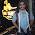## Inversions of double slider crank chain

Inversions of double slider crank chain: In this article we will learn about Inversions of double slider crank chain. In Inversions of double slider crank chain there are three important double slider crank chain. they are explained as follows.

There are three important double Slider Crank Chain are:

1.  Elliptical trammels
2.  Scotch yoke Mechanism
3. Oldham's Coupling

This type of   instrument is used for  drawing ellipse. By fixing the slotted plate (link 4) the inversion is obtained. the two straight grooves has a single link 4 which is cut in it at right angle to each other. With the sliding pair with link 4 there are two sliders link 1 & 2. The link 2 is a bar which forms turning pair with link 1 and link 3.
With respective to the grooves link 1&3 it slides along there, any point on the link 2 such as p traces out an ellipse on the surface of link 4,There are the semi-major axis and semi- minor axis of the ellipse respectively with the Consideration on OB & OQ. This can be proved as follows

Let us take  a horizontal and vertical axes as CX & CY and let the link AB is inclined at an angle C with horizontal, as shown in figure. Now the coordinates of the point O on the link AB will be

This is the equation of the ellipse . Hence the path traced by an point O is an ellipse whose semi major axis is OB and semi- minor axis is OA.

This is the Equation of a circle whose radius is OB. hence O is the mid-point of Link BA, It will Trace a Circle.

## 2.  Scotch yoke Mechanism:

This mechanism is used for converting the rotary motion in to a reciprocating motion. These type of inversion is obtained by fixing either the link 1 or link 3.We can observe In this figure the link 1, Is fixed. In this mechanism, Whit the help of link 2 this  rotates about the center B,and the link 4 reciprocates. In this fixed link 1 guides the frame.

## 3. Oldham's Coupling:

Old ham's coupling is the one of most used coupling method to rotate the connecting shafts with equal speed, this is done by parallel shaft connection which are at very less distance apart.
The shafts are coupled in such a way that if one shaft rotates, the other shaft rotates with same speed.By fixing the link 2  to obtain the Inversion, as shown in figure. The shaft to be connected have two flanges (link 1 and link 3) rigidly fastened at there ends by forging. This helps to transfer the rotation speed of driving shafts makes equal/same rotational speed to driven shaft.
The link 1 and link 3 form turning pair with link 2. these flanges have there Diametrical slot cut in there inner face as shown in figure.

Oldham's Coupling

1.2.3.